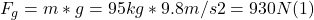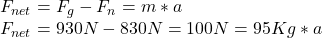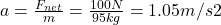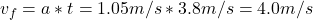## Henry, whose mass is 95 kg, stands on a bathroom scale in an elevator. The scale reads 830 N for the first 3.8 s after the elevator starts m

Question

Henry, whose mass is 95 kg, stands on a bathroom scale in an elevator. The scale reads 830 N for the first 3.8 s after the elevator starts moving, then 930 N for the next 3.8 s. What is the elevator’s speed 7.6 s after starting?

in progress 0
2 weeks 2021-09-05T08:18:34+00:00 1 Answers 0 views 0

v= 4.0 m/s

Explanation:

• When standing on the bathroom scale within the moving elevator, there are two forces acting on Henry’s mass: Normal force and gravity.
• Gravity is always downward, and normal force is perpendicular to the surface on which the mass is located (the bathroom scale), in upward direction.
• Normal force, can adopt any value needed to match the acceleration of the mass, according to Newton’s 2nd Law.
• Gravity (which we call weight near the Earth’s surface) can be  calculated as follows:• According to Newton’s 2nd Law, it must be met the following condition:• As the gravity is larger than normal force, this means that the acceleration is downward, so, we choose this direction as the positive.
• Solving for a, we get:• We can find the speed after the first 3.8 s (assuming a is constant), applying the definition of acceleration as the rate of change of velocity:• Now, if during the next 3.8 s, normal force is 930 N (same as the weight), this means that both forces are equal each other, so net force is 0.
• According to Newton’s 2nd Law, if net force is 0, the object  is either or at rest, or moving at a constant speed.
• As the elevator  was moving, the only choice is that it is moving at  a constant speed, the same that it had when the scale was read for the first time, i.e., 4 m/s downward.